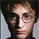12060 vizualizações
12060
The RSI is a very popular indicator that follows price activity.
It calculates an average of the positive net changes, and an average
of the negative net changes in the most recent bars, and it determines
the ratio between these averages. The result is expressed as a number
between 0 and 100. Commonly it is said that if the RSI has a low value,
for example 30 or under, the symbol is oversold. And if the RSI has a
high value, 70 for example, the symbol is overbought.
```////////////////////////////////////////////////////////////
//  Copyright by HPotter v1.0 19/05/2014
// The RSI is a very popular indicator that follows price activity.
// It calculates an average of the positive net changes, and an average
// of the negative net changes in the most recent bars, and it determines
// the ratio between these averages. The result is expressed as a number
// between 0 and 100. Commonly it is said that if the RSI has a low value,
// for example 30 or under, the symbol is oversold. And if the RSI has a
// high value, 70 for example, the symbol is overbought.
////////////////////////////////////////////////////////////
study(title="Strategy RSI", shorttitle="Strategy RSI", overlay = true )
Length = input(12, minval=1)
Oversold = input(30, minval=1)
Overbought = input(70, minval=1)
xRSI = rsi(close, Length)
pos =	iff(xRSI > Overbought, 1,
iff(xRSI < Oversold, -1, nz(pos, 0)))
barcolor(pos == -1 ? red: pos == 1 ? green : blue)
```
DONATE/TIP

BTC: 3FKWwtaYrf5NHZzaCi1fyAfQj7XSgtyCqeWARNING:
This script to change bars color in RED if position is short, and GREEN if position is long. This colors not equals bar color.
Resposta